# PLC Contacts & Coils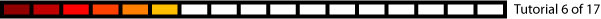The most common PLC programming language is Ladder Logic.  They consist of a series of electrical drawings that control how the PLC functions.  We’ll go through the basics of Ladder Logic starting with Coils and Contacts.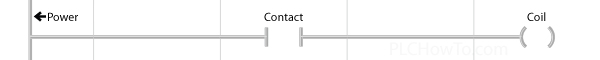Contacts stand between power and Coils, asking questions.  If the answer to the question is true, then power is allowed to flow through the contact, if not the path is blocked.

List of typical Contacts: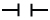Normally Open asks if a bit is = 1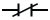Normally Closed asks if a bit is = 0Rising Edge asks if a bit just turned from 0 to 1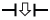Falling Edge asks if a bit just turned from 1 to 0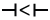Less Than asks if Value A is less than Value B.Greater Than asks if Value A is greater than Value B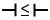Less Than or Equal asks if Value A is less than or equal to Value B.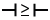Greater Than or Equal asks if Value A is greater than or equal to Value B.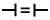Equal asks if Value A is equal to Value B.Not Equal asks if Value A is not equal to Value B.

Note: We’re about to use the & (AND) and | (OR) symbol.  They are discussed on the Math page.

Contacts placed side by side to form AND statements.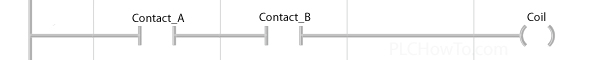Example: Coil gets power if: Contact_A & Contact_B

Contacts placed one on top of each other to form OR statements.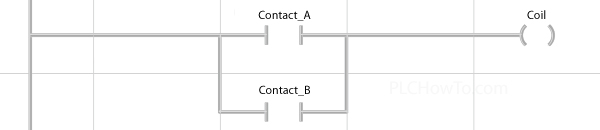Example: Coil gets power if: Contact_A | Contact_B

AND and OR statements may be combined to form complex expressions.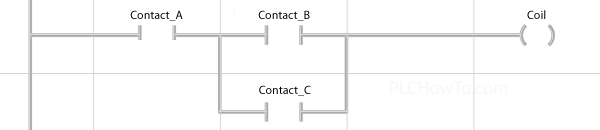Example: Coil gets power if: Contact_A & (Contact_B | Contact_C)

### Coils

Each Coil is tied to a specific Bit of data.  Here are the standard Coil Types and what they to the specified Bit.

• Out: If the Coil is powered, the bit is assigned a 1.  If not it’s assigned a 0.
• Set: If the Coil is powered, the bit is assigned a 1.  If not, the value remains whatever it was.
• Reset: If a Coil is powered, the bit is assigned a 0.  If not, the value remains whatever it was.
Contacts and coils are often associated with the physical Inputs & Outputs on the PLC.
If  a contact is tied to an Input, then when the power at the input changes it’s contact value in the Ladder Logic will Change.  If a coil is tied to an output and the coil changes it’s value, then the voltage (or contact state) at the output will change.

Functions:  As Ladder Logic has evolved, additional functionality has been added.  In many Ladder Logic packages the Coil location can be replaced by function blocks that do specific tasks.

The Math function has already been discussed.  The tutorials that follow focus on other common functions.
Functions we’ll go over: